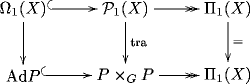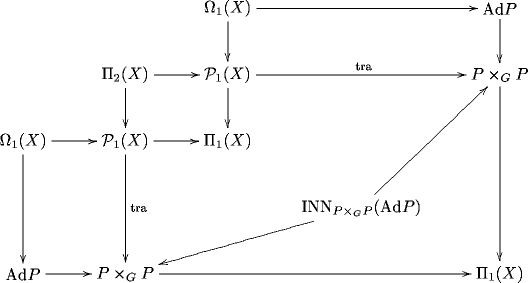## June 20, 2007

### Curvature, the Atiyah Sequence and Inner Automorphisms

#### Posted by Urs SchreiberI am still trying to better understand $n$-curvature and its relation to inner automorphism $(n+1)$-groups.

A while ago David Roberts emphasized that the parallel transport functor $\mathrm{tra} : P_1(X) \to G\mathrm{Tor}$ of a principal $G$-bundle $P \to X$ with connection can be thought of as inducing a morphism of short exact sequences of groupoids: from the sequence of path groupoids of $X$ to the integrated Atiyah sequence of $P$.

Here I take a fresh look at the curvature 2-functor of a parallel transport 1-functor from this point of view, emphasizing the role played by inner automorphisms in this construction.

Let $P_1(X)$ be the groupoid of thin homotopy classes of paths and$\Pi_1(X)$ is the fundamental groupoid.

Then we have a short exact sequence of path groupoids $\Omega_1(X) \to P_1(X) \to \Pi_1(X) \,.$ $\Omega_1(X)$ is the groupoid of closed paths (loops).

On the other hand, the integrated Atiyah sequence of the principal bundle $P$ is the short exact sequence $\mathrm{Ad} P \to P \times_G P \to \Pi_1(X) \,.$ (Here I am for simplicitly assuming that $X$ is simply connected, so that $\Pi_1(X)$ is the codiscrete grupoid over $X$. If $X$ is not simply connected there is a relatively straightforward generalization of everything in sight.)

For any connection on $P$, its parallel transport is a functor $\mathrm{tra} : P_1(X) \to P \times_G P \hookrightarrow G\mathrm{Tor} \,.$

This extends to a morphism of exact sequences of groupoids$\;\;$ (A)

One crucial point is that the groupoids in the middle of the sequences act by conjugation – hence by “inner” automorphisms – on those on the left.

This allows us to turn the parallel transport 1-functor $\mathrm{tra}$ into a functor $\mathrm{Ad}\circ \mathrm{tra} : P_1(X) \to \mathrm{INN}_{P \times_G P}(\mathrm{Ad} P) \,.$ This functor tells us how the monodromy groups of the original functor get transformed as we conjugate loops by paths.The 2-groupoid $\mathrm{INN}_{P \times_G P}(\mathrm{Ad} P)$ is codiscrete at top level (all its Hom-categories are codiscrete). This is the 2-groupoid that the curvature 2-functor of $\mathrm{tra}$ takes values in. Codiscreteness of $\mathrm{INN}_{P \times_G P}(\mathrm{Ad} P)$ then induces the Bianchi identity on curvature.

To see this – and that’s the point of this discussion here – we pull back the sequences (A) along themselves to obtainHere the fundamental 2-groupoid $\Pi_2(X)$ appears in its incarnation where 1-morphisms are taken to be thin homotopy classes of paths. (And now I am even assuming that $X$ is 2-connected, for simplicity. Otherwise there is a more or less obvious way to adapt the entire construction.)

Then the two nested pullbacks give us a unique canonical factorization morphism. That’s our curvature 2-functorSo by regarding the connection as a morphism from the path sequence of the base to the Atiyah sequence of the bundle, also the discussion of curvature becomes a little more compelling.

More details in the above mentioned notes.

Posted at June 20, 2007 4:23 PM UTC

TrackBack URL for this Entry:   https://golem.ph.utexas.edu/cgi-bin/MT-3.0/dxy-tb.fcgi/1329

### Re: Curvature, the Atiyah Sequence and Inner Automorphisms

Here is yet another way to see the same. This is closely analogous the the discussion of (twisted) vector bundles as morphisms into 2-vector transport 2-functors which I gave here.

Let $GrpSpc$ be the 2-category whose

- objects are groups

- morphisms $S : G \to H$ are left $G$- right $H$-spaces

- 2-morphisms are maps of $G$-$H$-spaces, commuting with both actions.

This plays the analogous role for principal 1- and 2-bundles as $\mathrm{Bimodules}$ does for vector 1- and 2-bundles.

Curvature of a principal 2-bundles with connection is a 2-functor $\mathrm{curv} : P_2(X) \to \mathrm{GrpSpc}$ which is such that it trivializes in the sense that there is a morphism $\mathrm{TRA} : I \to \mathrm{curv}$ into it.

Here $I : P_2(X) \to \mathrm{GrpSpc}$ is the 2-functor which sends everything to the identity on the trivial group.

The component map of $\mathrm{TRA}$ – a functor on $P_1(X)$ – gives the parallel transport $\mathrm{tra} : P_1(X) \to G\mathrm{Tor}$.

To see this, notice that $\mathrm{curv}$ will send any surface

$\array{ & \nearrow \searrow^{\gamma} \\ x &\Downarrow^\Sigma& y \\ & \searrow\nearrow_{\gamma^\prime} }$ to $\array{ & \nearrow && \searrow^{\mathrm{Aut}(P_x)_{\mathrm{Ad}(\mathrm{tra}(\gamma))}} \\ \mathrm{Aut}(P_x) & & \Downarrow^{\mathrm{tra}(\gamma^{-1}\circ \gamma')}& & \mathrm{Aut}(P_y) \\ & \searrow && \nearrow_{{\mathrm{Aut}(P_x)_{\mathrm{Ad}(\mathrm{tra}(\gamma'))}}} } \,.$

(Here a group $G$ with an automorphism $\alpha$ in the subscript, $G_\alpha$ denotes $G$ as a $G$-$G$ space with the obvious left and the $\alpha$-twisted right action.)

Here $P_x$, $P_y$ are the fibers of a principal $G$-bundle over $X$ and $\mathrm{Aut}(P_x)$ is their automorphism group in the category of $G$-spaces. This is canonically isomorphic to the group $\mathrm{Ad}P_x := P_x \times_G G$ which appeared a lot in the above entry.

Notice how the fact that the 2-functor $\mathrm{curv}$ admits a “2-section” $I \to \mathrm{curv}$ forces it to takes values in a sub-2-category of $\mathrm{GrpSpc}$ which in the above entry I denoted $\mathrm{INN}_{P \times_G P}(\mathrm{Ad}P) \,.$

This is equivalent to $\mathrm{INN}(G)$ and hence, in particular, trivializable.

While $\mathrm{curv}$ is hence trivializable, it is the choice of the trivialization, $\mathrm{TRA}$, which encodes the interesting information:

one and the same curvature 2-functor may be trivialized by several non-equivalent morphisms $\mathrm{TRA}$ and $\mathrm{TRA}'$.

I am emphasizing this point since David Corfield was wondering about this here. The above is one example for the answer which I gave here.

Posted by: urs on June 22, 2007 2:22 PM | Permalink | Reply to this

### Re: Curvature, the Atiyah Sequence and Inner Automorphisms

Here is a pdf version of the above sketch of how curvature is a principal 2-transport trivialized by its parallel 1-transport $\mathrm{tra} : I_G \stackrel{\sim}{\to} \mathrm{curv} \,.$

Posted by: urs on June 26, 2007 9:25 PM | Permalink | Reply to this
Read the post The inner automorphism 3-group of a strict 2-group
Weblog: The n-Category Café
Excerpt: On the definition and construction of the inner automorphism 3-group of any strict 2-group, and how it plays the role of the universal 2-bundle.
Tracked: July 4, 2007 12:52 PM
Read the post The G and the B
Weblog: The n-Category Café
Excerpt: How to get the bundle governing Generalized Complex Geometry from abstract nonsense and arrow-theoretic differential theory.
Tracked: August 25, 2007 9:18 PM
Read the post n-Curvature, Part III
Weblog: The n-Category Café
Excerpt: Curvature is the obstruction to flatness. Believe it or not.
Tracked: October 16, 2007 10:51 PM

Post a New Comment